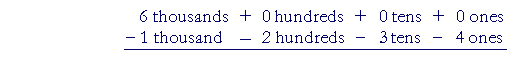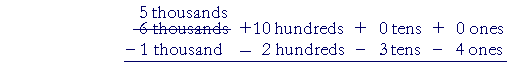S k i l l
i n
A R I T H M E T I C

Lesson 8

# SUBTRACTING WHOLE NUMBERS AND DECIMALS

In this Lesson, we will answer the following:

12 − 9 = ?

The problem of subtraction is to name the number we must add to the smaller number so that their sum will equal the larger number.  How could you know that 12 minus 9 is 3?  Because you know that 9 plus 3 is 12. That is, you know elementary addition.

We will now present two methods of written subtraction.  One is the familiar subtraction by regrouping, or borrowing.  The other is the less familiar but simpler method of adding to both numbers.  It is taught in many countries, and is based on the following property of subtraction:

If we add the same number to both the larger and the smaller, the difference will not change.

10 − 8 = 12 − 10.

We illustrated that in the previous Lesson.  We will present that method first.

(For subtraction by regrouping, or borrowing, see below.)

Subtraction by adding to both numbers

Problem.  The town of Huntley has a population of 8,345.  The neighboring town of Jasper has a population of 5,872.  How many more people live in Huntley?

Solution.  What must we add to the population of Jasper to equal the population of Huntley?  That is a subtraction problem.

 8,345 − 5,872

First, we will state the rule for doing it; then we will analyze why.

 1. How do we subtract by adding to both numbers? − 8,345 − 5,872 − 2,473 Write the smaller number under the larger, taking care to align the same units. Then, starting with the ones on the right, subtract each digit on the bottom from the corresponding digit on top. When the bottom digit is greater, consider the top digit increased by 10. Upon doing that, add 1 to the next bottom digit.

Begin:

 8,345 − 5,872 2,473

"2 from 5 is 3."

No problem.

Now, 7 is greater than 4. Therefore, say 7 from 14:

"7 from 14 is 7"

—and add 1 to 8:

"9 from 13 is 4."

Since we called the top number 13, add 1 to 5:

"6 from 8 is 2."

To understand why we do this, here are the expanded forms:

 8 thousands + 3 hundreds + 4 tens + 5 ones − 5 thousands − 8 hundreds − 7 tens − 2 ones 2 thousands + 4 hundreds + 7 tens + 3 ones

2 ones from 5 ones are 3 ones.   No problem.

Now we cannot take 7 tens from 4 tens.  Therefore we will consider 10 tens added to the 4 tens, and we will take 7 from 14.  To compensate, we will add 1 hundred to 8 -- the next bottom number -- making it 9. Because 1 hundred is equal to the 10 tens we added to 4.  (Lesson 2.)

Continuing:

9 hundreds from 13 hundreds are 4 hundreds.

6 thousands from 8 thousands are 2 thousands.

 Example 1. 5,312 − 2,579 2,733

"9 from 12 is 3."

"8 from 11 is 3."

"6 from 13 is 7."

"3 from 5 is 2."

The top digits  5312  never change.  That is the simplicity of this method.  Only a bottom digit might change.

Finally, since subtracting is finding what number to add to the smaller number, the student should always check the answer by adding.

3 + 9 is 12.   3 + 7 is 10, plus 1 is 11.   And so on.

 Example 2. 6,000 −1,926 4,074

"6 from 10 is 4."

"3 from 10 is 7."

"10 from 10 is 0."

"2 from 6 is 4."

Example 3.    30.21 − .86

Solution.   The smaller number is .86   Write it on the bottom, and align the decimal points:
 30.21 −.86 29.35

"6 from 11 is 5."

"9 from 12 is 3."

"1 from 10 is 9."

"1 from 3 is 2."

Example 4.   2.1 − .867

Solution.   .867 is the smaller number.  Write it on the bottom.  Align the decimal points.

Note that we must add 0's onto the right of 2.1 (Lesson 3).  Both numbers must have the same number of decimal digits.On checking:  .867 plus 1.233 is 2. 100.

Example 5.    Subtract  .698  from  5

Solution.  This problem means

5 − .698

.698 is the smaller number.  Write it below 5.000:Subtraction by regrouping

First, here is a simple example where we do not have to regroup, or borrow:

 48 = 4 tens + 8 ones − 12 = − 1 ten  − 2 ones 36 = 3 tens + 6 ones

2 ones from 8 ones are 6 ones.

1 ten from 4 tens are 3 tens.

(Lesson 2)

In practice, simply say:

 48 − 12 36

"2 from 8 is 6.  1 from 4 is 3."

But say that we have

 42 = 4 tens + 2 ones − 18 = − 1 ten  − 8 onesWe cannot take 8 ones from 2 ones. We need more ones. Therefore we will "borrow" 1 of the tens and regroup it as 10 ones:The top number is now 3 tens plus 12 ones.

8 ones from 12 ones are 4 ones.

1 ten from 3 tens are 2 tens.

In practice, say:"8 from 12 is 4." (Because 8 plus 4 is 12.)  "1 from 3 is 2."

 2. How do we subtract by regrouping, or borrowing?When the digit in the bottom number is larger (8 is larger than 2), decompose 1 unit of higher place value into 10 units of the next lower place value, and regroup with those lower units.We cannot take 9 from 4.Therefore, we will borrow 1 from 3 -- making it 2 -- and regroup that 1 with 4.

"9 from 14 is 5."  Because 9 plus 5 is 14.

Next, we cannot take 8 from 2.  So we will borrow 1 from 8, making it 7 ---- and regroup that 1 with 2.

"8 from 12 is 4.  1 from 7 is 6."

We can always check the difference  645  by adding it to the smaller number.  "5 plus 9 is 14.  4 plus 8 is 12, plus 1 is 13." And so on.

In the interest of skill, the student should try not to write the crossings out or the regrouped numbers.  Those steps should be practiced mentally. The answer can always be checked by adding.

In other words, with practice the student should be able to say:"9 from 14 is 5."

"8 from 12 is 4."

"1 from 7 is 6."Here is the expanded form:We cannot take 4 ones from 0 ones, and we cannot decompose 0 tens. We must move left and start decomposing at the first place that is not 0, namely 6.

6 is in the thousands place.  We will decompose 1 of those thousands into 10 hundreds, and regroup them with 0 hundreds.We now have 5 thousands and 10 hundreds.

Now we will decompose 1 of the 10 hundreds into 10 tens.Finally, we can decompose 1 of the 10 tens into 10 ones.That completes the decomposing and regrouping.  The subtraction follows easily :The answer is 4,766.

The problem will appear, of course, like this:With practice, the student should be able to say,

"4 from 10 is 6."

"3 from 9 is 6."

"2 from 9 is 7."

"1 from 5 is 4."

Again, we can always check the answer by adding.

(Please compare the simplicity of adding to both numbers.)

Example 8.  Alignment.   68.27 − 3

Answer.   3 is 3 ones.  We must subtract it from 8 ones.  The answer is 65.27.

As in addition, we must align the digits that have the same place value.

Example 9.   Your checking account has a balance of $310.12, and you write a check for$45.24.  You then make a deposit of 126.75, and you write another check for \$22.50.  What is your present balance?

Answer.   Each time you write a check, you must subtract.  And each time you make a deposit, you add.  Here then is the sequence of subtractions and additions:

 310.12 Opening balance −  45.24 Check 264.88 + 126.75 Deposit 391.63 −  22.50 Check 369.13 Present balance

Please "turn" the page and do some Problems.

or

Continue on to the next Lesson.